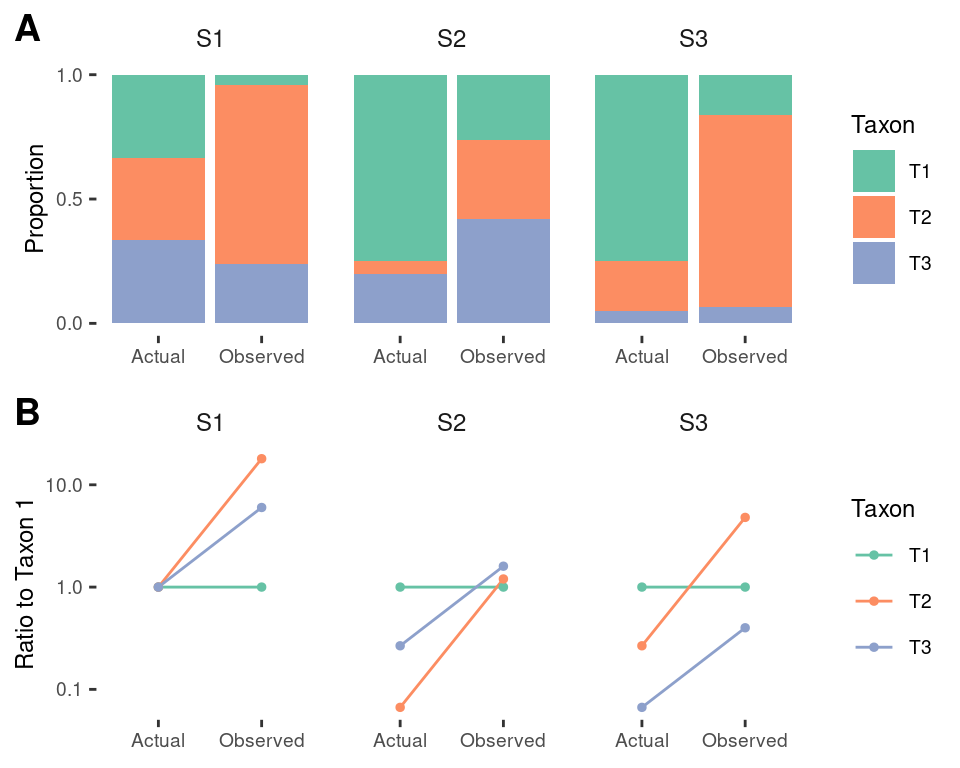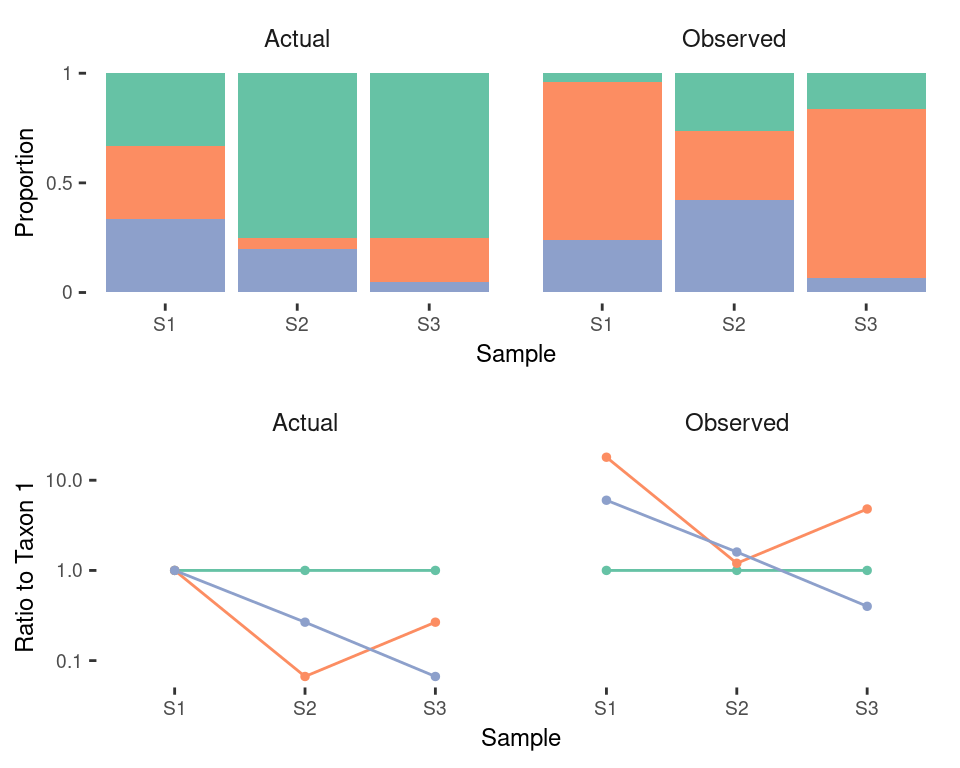# R setup

library(here)
library(tidyverse)
library(ggthemes)
# library(cowplot)
# library(ggbeeswarm)
library(metacal)
# This package
devtools::load_all(here())
base_theme <- theme_tufte() +
theme(
text = element_text(size=9, family = ""),
legend.position = "none"
)
base_theme0 <- theme_grey() +
theme(
panel.grid.major = element_blank(),
panel.grid.minor = element_blank(),
panel.background = element_blank()
)
tax_theme <- theme(
axis.text.x = element_text(angle = 45, hjust = 1, vjust = 1),
axis.title.x = element_blank())

update_geom_defaults("point", list(size = 1))
update_geom_defaults("text", list(size = 2.5))
update_geom_defaults("hline", list(color = "grey"))

colors.taxa <- c(
T1 = "#66c2a5",
T2 = "#fc8d62",
T3 = "#8da0cb"
)

# Example: three-species communities

We will consider the three taxa in the main text Figures 1 and 2, with bias equal to (1, 18, 6). We consider three samples; samples S1 and S2 in the main text, and a third sample S3 that switches the abundance of the second and third taxa in sample S3. First we create a data frame with the actual compositions and the bias.

tb <- tibble(
Taxon = c("T1", "T2", "T3"),
Bias = c(1, 18, 6),
S1 = c(1, 1, 1),
S2 = c(1, 1/15, 4/15),
S3 = c(1, 4/15, 1/15),
)
print(tb)
## # A tibble: 3 x 5
##   Taxon  Bias    S1     S2     S3
##   <chr> <dbl> <dbl>  <dbl>  <dbl>
## 1 T1        1     1 1      1
## 2 T2       18     1 0.0667 0.267
## 3 T3        6     1 0.267  0.0667

Note that we have expressed bias and the relative abundances as relative to taxon 1. Then, we gather these relative abundances into a tidy form, use the bias to calculate the observed abundances, and also obtain the proportions from the relative abundances.

tb <- tb %>%
gather("Sample", "Actual", S1:S3) %>%
mutate(Observed = Actual * Bias) %>%
gather("Type", "Abundance", Actual, Observed) %>%
mutate_by(c(Sample, Type), Proportion = close_elts(Abundance)) %>%
select(Sample, Taxon, Bias, everything())
tb
## # A tibble: 18 x 6
##    Sample Taxon  Bias Type     Abundance Proportion
##    <chr>  <chr> <dbl> <chr>        <dbl>      <dbl>
##  1 S1     T1        1 Actual      1          0.333
##  2 S1     T2       18 Actual      1          0.333
##  3 S1     T3        6 Actual      1          0.333
##  4 S2     T1        1 Actual      1          0.75
##  5 S2     T2       18 Actual      0.0667     0.05
##  6 S2     T3        6 Actual      0.267      0.2
##  7 S3     T1        1 Actual      1          0.75
##  8 S3     T2       18 Actual      0.267      0.2
##  9 S3     T3        6 Actual      0.0667     0.05
## 10 S1     T1        1 Observed    1          0.04
## 11 S1     T2       18 Observed   18          0.72
## 12 S1     T3        6 Observed    6          0.24
## 13 S2     T1        1 Observed    1          0.263
## 14 S2     T2       18 Observed    1.2        0.316
## 15 S2     T3        6 Observed    1.6        0.421
## 16 S3     T1        1 Observed    1          0.161
## 17 S3     T2       18 Observed    4.8        0.774
## 18 S3     T3        6 Observed    0.4        0.0645

## Error in ratios vs. error in proportions

The proportions used to label Figure 2:

tb0 <- tb %>%
select(-Abundance) %>%
arrange(Sample, Taxon)
tb0 %>%
knitr::kable(digits = 2, format = "pandoc")
Sample Taxon Bias Actual Observed
S1 T1 1 0.33 0.04
S1 T2 18 0.33 0.72
S1 T3 6 0.33 0.24
S2 T1 1 0.75 0.26
S2 T2 18 0.05 0.32
S2 T3 6 0.20 0.42
S3 T1 1 0.75 0.16
S3 T2 18 0.20 0.77
S3 T3 6 0.05 0.06

We can view the measurement error across the three samples with bar plots, as done in main text Figure 2, and also in terms of the ratios to Taxon 1:

p.props <- ggplot(tb, aes(x = Type, y = Proportion, fill = Taxon)) +
geom_bar(stat = "identity") +
scale_fill_manual(values = colors.taxa) +
facet_wrap(~Sample) +
scale_y_continuous(breaks = c(0, 0.5, 1)) +
base_theme +
theme(strip.text = element_text(size = 9), axis.title.x = element_blank(),
legend.position = "right")
p.ratios <- ggplot(tb, aes(x = Type, y = Abundance, color = Taxon)) +
geom_path(aes(group = Taxon)) +
geom_point() +
facet_wrap(~Sample) +
scale_y_log10() +
scale_color_manual(values = colors.taxa) +
base_theme +
labs(y = "Ratio to Taxon 1") +
theme(strip.text = element_text(size = 9), axis.title.x = element_blank(),
legend.position = "right")
cowplot::plot_grid(p.props, p.ratios, ncol = 1, align = "v", axis = "lr",
labels = c("A", "B"))From this figure, we can see that the error in the proportions differs for the three samples, but the error in ratios is consistent.

Calculate the compositional error and the fold-error in proportions:

err <- tb %>%
select(-Abundance) %>%
arrange(Sample, Taxon) %>%
mutate(Error = Observed / Actual) %>%
mutate_by(Sample,
Error.T1 = Error / Error,
)
err %>%
knitr::kable(digits = 2, format = "pandoc")
Sample Taxon Bias Actual Observed Error Error.T1
S1 T1 1 0.33 0.04 0.12 1
S1 T2 18 0.33 0.72 2.16 18
S1 T3 6 0.33 0.24 0.72 6
S2 T1 1 0.75 0.26 0.35 1
S2 T2 18 0.05 0.32 6.32 18
S2 T3 6 0.20 0.42 2.11 6
S3 T1 1 0.75 0.16 0.22 1
S3 T2 18 0.20 0.77 3.87 18
S3 T3 6 0.05 0.06 1.29 6

The Error column shows the fold error in the Proportions, while the Error.T1 column divides this by the Error of taxon T1 in each sample and equals the Bias relative to T1. We can see that the fold-error error in proportions of a given taxon varies across samples, but when divided by the fold-error in taxon T1, is consistent.

The sample mean efficiencies are

sme <- err %>%
group_by(Sample) %>%
summarize(SME = sum(Actual * Bias) / sum(Actual))
sme
## # A tibble: 3 x 2
##   Sample   SME
##   <chr>  <dbl>
## 1 S1      8.33
## 2 S2      2.85
## 3 S3      4.65

Check that the observed proportions are given by the equation from the main text—

err0 <- left_join(err, sme, by = "Sample")
err0 %>%
mutate(Observed0 = Actual * Bias / SME) %>%
{all.equal(.$Observed, .$Observed0)}
##  TRUE

## Analysis of compositional differences between samples

We get very different pictures comparing the three samples based on the Actual or the Observed proportions.

p.props <- ggplot(tb, aes(x = Sample, y = Proportion, fill = Taxon)) +
geom_bar(stat = "identity") +
facet_wrap(~Type) +
scale_y_continuous(breaks = c(0, 0.5, 1), labels = c(0, 0.5, 1)) +
scale_fill_manual(values = colors.taxa) +
base_theme +
theme(strip.text = element_text(size = 9))

p.ratios <- ggplot(tb, aes(x = Sample, y = Abundance, color = Taxon)) +
geom_path(aes(group = Taxon)) +
geom_point() +
facet_wrap(~Type) +
scale_y_log10() +
scale_color_manual(values = colors.taxa) +
base_theme +
labs(y = "Ratio to Taxon 1") +
theme(strip.text = element_text(size = 9))

cowplot::plot_grid(p.props, p.ratios, ncol = 1)The lower panel shows that the change in ratios between samples is consistent whether using the actual and observed profiles.

From this figure, it is apparent that Sample S1 is the most even and thus has the highest diversity by any metric that values evenness (such as the Shannon or Inverse Simpson indices), but but sample S2 is observed to be the most even and thus to have the highest diversity.

We can also see that Samples S1 and S2 are more similar compositionally by the Bray-Curtis (BC) community similarity, but that samples S1 and S3 appear to be the most similar. This is easy to see because BC similarity, when applied to proportions, simply sums up the amount of shared proportion of each taxon.

B <- c(1, 18, 6)
A1 <- c(1, 1, 1)
A2 <- c(1, 1/15, 4/15)
A3 <- c(1, 4/15, 1/15)
O1 <- close_elts(A1 * B)
O2 <- close_elts(A2 * B)
O3 <- close_elts(A3 * B)

The BC index gives a different clustering when applied to the actual and observed compositions.

# Actual
xydist(A1, A2, method = "bray")
##  0.4166667
xydist(A1, A3, method = "bray")
##  0.4166667
xydist(A2, A3, method = "bray")
##  0.15
# Observed
xydist(O1, O2, method = "bray")
##  0.4042105
xydist(O1, O3, method = "bray")
##  0.1754839
xydist(O2, O3, method = "bray")
##  0.4584041

The Aitchison distances are invariant to bias, although sample 1 is equidistant from samples 2 and 3, so clustering isn’t possible in this toy example.

# Actual
xydist(A1, A2, method = "aitchison")
##  1.915062
xydist(A1, A3, method = "aitchison")
##  1.915062
xydist(A2, A3, method = "aitchison")
##  1.960516
# Observed
xydist(O1, O2, method = "aitchison")
##  1.915062
xydist(O1, O3, method = "aitchison")
##  1.915062
xydist(O2, O3, method = "aitchison")
##  1.960516

## Taxonomic aggregation

The theoretical bias invariance of ratio-based analyses does not hold under the common practice of taxonomically agglomerating abundances prior to analysis. For example, suppose the first two taxa are in phylum P1 and the third taxon is in phylum P2.

tb1 <- tb %>%
mutate(Phylum = ifelse(Taxon < "T3", "P1", "P2")) %>%
group_by(Sample, Type, Phylum) %>%
summarize(Proportion = sum(Proportion)) %>%
ungroup
tb1
## # A tibble: 12 x 4
##    Sample Type     Phylum Proportion
##    <chr>  <chr>    <chr>       <dbl>
##  1 S1     Actual   P1         0.667
##  2 S1     Actual   P2         0.333
##  3 S1     Observed P1         0.76
##  4 S1     Observed P2         0.24
##  5 S2     Actual   P1         0.8
##  6 S2     Actual   P2         0.2
##  7 S2     Observed P1         0.579
##  8 S2     Observed P2         0.421
##  9 S3     Actual   P1         0.95
## 10 S3     Actual   P2         0.05
## 11 S3     Observed P1         0.935
## 12 S3     Observed P2         0.0645

Consider the ratio of phylum 1 to phylum 2,

tb1 <- tb1 %>%
mutate(Ratio = P1 / P2) %>%
select(-P1, -P2) %>%
tb1
## # A tibble: 2 x 4
##   Type        S1    S2    S3
##   <chr>    <dbl> <dbl> <dbl>
## 1 Actual    2     4     19.0
## 2 Observed  3.17  1.38  14.5

From Community 1 to Community 2, the ratio of P1 to P2 increases by a factor of 4/2 = 2x but is observed to decrease by factor of 1.38/3.17 = 0.44x.

# Session info

sessionInfo()
## R version 3.6.0 (2019-04-26)
## Platform: x86_64-pc-linux-gnu (64-bit)
## Running under: Arch Linux
##
## Matrix products: default
## BLAS:   /usr/lib/libblas.so.3.8.0
## LAPACK: /usr/lib/liblapack.so.3.8.0
##
## Random number generation:
##  RNG:     Mersenne-Twister
##  Normal:  Inversion
##  Sample:  Rounding
##
## locale:
##   LC_CTYPE=en_US.UTF-8       LC_NUMERIC=C
##   LC_TIME=en_US.UTF-8        LC_COLLATE=en_US.UTF-8
##   LC_MONETARY=en_US.UTF-8    LC_MESSAGES=en_US.UTF-8
##   LC_PAPER=en_US.UTF-8       LC_NAME=C
##  LC_MEASUREMENT=en_US.UTF-8 LC_IDENTIFICATION=C
##
## attached base packages:
##  stats     graphics  grDevices utils     datasets  methods   base
##
## other attached packages:
##   cowplot_0.9.99            ggbeeswarm_0.6.0
##   bindrcpp_0.2.2            rmarkdown_1.13
##   BiasManuscript_0.0.0.9000 testthat_2.1.1
##   ggthemes_4.2.0            forcats_0.4.0
##   stringr_1.4.0             dplyr_0.8.1
##  tidyr_0.8.3               tibble_2.1.3
##  ggplot2_3.1.1             tidyverse_1.2.1
##  here_0.1                  nvimcom_0.9-82
##  usethis_1.5.0
##
## loaded via a namespace (and not attached):
##   modelr_0.1.4      assertthat_0.2.1  highr_0.8
##   vipor_0.4.5       cellranger_1.1.0  yaml_2.2.0
##  remotes_2.0.4     sessioninfo_1.1.1 pillar_1.4.1
##  backports_1.1.4   lattice_0.20-38   glue_1.3.1
##  digest_0.6.19     rvest_0.3.4       colorspace_1.4-1
##  htmltools_0.3.6   plyr_1.8.4        pkgconfig_2.0.2
##  devtools_2.0.2    broom_0.5.2       haven_2.1.0
##  scales_1.0.0      processx_3.3.1    generics_0.0.2
##  withr_2.1.2       lazyeval_0.2.2    cli_1.1.0
##  vctrs_0.1.0       tidyselect_0.2.5  xfun_0.7